Adjusting a traverse (also known as balancing a traverse) is used to distributed the closure error back into the angle and distance measurements.

Summing the latitudes and departures for the raw field traverse:Equation E-1Figure E-1
Loop Traverse MisclosureEquation E-2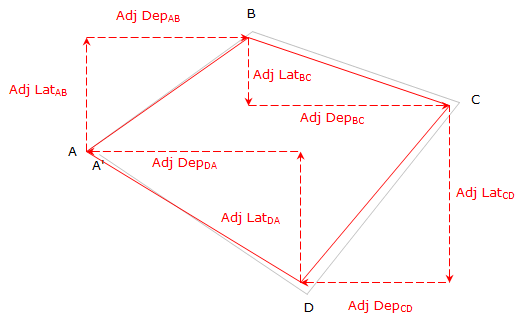Figure E-2

The condition for an adjusted traverse is that the adjusted Lats and Deps sum to 0.00. As with other survey adjustments, the method used to balance a traverse should reflect the expected error behavior and be repeatable. Table E-1 lists primary adjustment methods with their respective advantages and disadvantages.

 Table E-1 Method Premise Advantage Disadvantage Ignore Don't adjust anything. Simple; repeatable Ignores error Arbitrary Place error in one or more measurements Simple Not repeatable; ignores error behavior Compass Rule Assumes angles and distances are measured with equal accuracy so error is applied to each. Simple; repeatable; compatible with contemporary measurement methods. Treats random errors systematically Transit Rule Assumes angles are measured more accurately than distances; distances receive greater adjustment. Simple; repeatable; compatible with older transit-tape surveys. Treats random errors systematically; not compatible with contemporary measurement methods. Crandall Method Quasi-statistical approach. Angles are held and errors are statistically distributed into the distances. Allows some random error modeling; repeatable. Models only distance errors, not angle errors. Least squares Full statistical approach. Allows full random error modeling; repeatable; can mix different accuracy and precision measurements; provides measurement uncertainties. Most complicated method

The Compass Rule works sufficiently well for simple surveying projects and is the one we will apply.

## 2. Compass Rule

The Compass Rule (also known as the Bowditch Rule) applies a proportion of the closure error to each line.

For any line IJ, Figure E-3,Figure E-3 Adjusted Latitude and DepartureEquation E-3Equation E-4

The Compass Rule distributes closure error based on the proportion of a line's length to the entire distance surveyed.

## 3. Adjusted Length and Direction

Regardless of the adjustment method applied, changing a line's Lat and Dep will in turn change the length and direction of the line.Figure E-4 Adjusted Length and Direction

The adjusted length can be computed from the Pythagorean theorem:Equation E-5

Computing direction is a two-step process: (1) Determine β, the angle from the meridian to the line (2) Convert β into a direction based on the line's quadrant.

To determine β:Equation E-6

β falls in the range of -90° to +90°.

The sign on β indicates the direction of turning from the meridian: (+) is clockwise, (-) is counter-clockwise. The combined signs on the adjusted Lat and Dep will identify the line's quadrant.

Figure E-5 shows the quadrant and direction computation for the various mathematic combinations of the adjusted Lat and Dep:Figure E-5 Converting ß to a Direction

4. Examples

These examples are a continuation of those from the Latitudes and Departures chapter.

### a. Traverse with BearingsFigure E-6 Bearing Traverse Example

 Line Bearing Length (ft) Lat (ft) Dep (ft) AB S 68°05'35"W 472.68 -176.357 -438.548 BC N 19°46'00"W 216.13 +203.395 -73.093 CD N 45°55'20"E 276.52 +192.357 +198.651 DA S 54°59'15"E 382.24 -219.312 +313.065 sums: 1347.57 +0.083 +0.075 Distance Lat err too far N Dep err too far E

#### (1) Adjust the Lats and Deps

Setup Equation E-3:Line ABLine BCLine CDLine DACheck the closure condition

 Adjusted Line Lat (ft) Dep (ft) AB -176.386 -438.574 BC +203.382 -73.105 CD +192.340 +198.635 DE -219.336 +313.044 sums: 0.000 0.000 check check

A common mistake is to forget to negate Lat err and Dep err in the correction equations. If that happens, the closure condition will be twice what it originally was as the corrections were applied in the wrong direction.

#### (2) Compute adjusted lengths and directions

Use Equations E-5 and E-6 along with Figure E-5 to compute the new length and direction for each line.

Line AB

Adj Lat = -176.386 <- South
Adj Dep = -438.574 <- West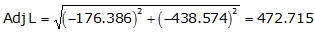Because it's the SW quadrant, Brng =S 68°05'27.4" W.

Line BC

Adj Lat = +203.382 <- North
Adj Dep = -73.105 <- WestBecause it's the NW quadrant, Brng = N 19°46'14.9" W

Line CD

Adj Lat = +192.340 <- North
Adj Dep = +198.635 <- EastBecause it's the NE quadrant, Brng = N 45°55'20.7" E

Line DA

Adj Lat = -219.336 <- South
Adj Dep = +313.044 <- East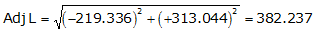Because it's the SE quadrant, Brng = S 54°58'58.0" E

 Adjusted Adjusted Line Lat (ft) Dep (ft) Length Bearing AB -176.386 -438.574 472.715 S 68°05'27.4" W BC +203.382 -73.105 216.122 N 19°46'14.9" W CD +192.340 +198.635 276.479 N 45°55'20.7" E DE -219.336 +313.044 382.237 S 54°58'58.0" E

### b. Traverse with AzimuthsFigure E-7 Azimuth Traverse Example

 Line Azimuth Length (ft) Lat (ft) Dep (ft) ST 309°05'38" 347.00 +218.816 -269.311 TU 258°34'22" 364.55 -72.226 -357.324 UV 128°04'44" 472.74 -291.560 +372.123 VS 60°21'26" 292.94 +144.885 +254.602 sums: 1477.23 -0.085 +0.090 Distance Lat err too far S Dep err too far E

#### (1) Adjust the Lats and Deps

Setup Equation E-3:Line STLine TULine UVLine VSCheck the closure condition

 Adjusted Line Lat (ft) Dep (ft) ST +218.836 -269.332 TU -72.205 -357.346 UV -291.533 +372.094 VS +144.902 +254.584 sums: 0.000 0.000 check check

#### (2) Compute adjusted lengths and directions

Use Equations E-5 and E-6 along with Figure E-5 to compute the new length and direction for each line.

Line ST

Adj Lat = +218.836 <- North
Adj Dep = -269.332 <- WestBecause it's in the NW quadrant: Az = 360°00'00"+(-50°54'20.4") =309°05'39.6"

Line TU

Adj Lat = -72.205 <- South
Adj Dep = -357.346 <- West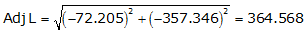Because it's in the SW quadrant: Az = 180°00'00"+(78°34'36.0") = 258°34'36.0"

Line UV

Adj Lat = -291.533 <- South
Adj Dep = +372.094 <- EastBecause it's in the SE quadrant: Az = 180°00'00"+(-51°55'17.6") = 128°04'42.4"

Line VS

Adj Lat = +144.902 <- North
Adj Dep = +254.584 <- EastBecause it's in the NE quadrant: Az = 60°21'09.7"

 Adjusted Adjusted Line Lat (ft) Dep (ft) Length (ft) Azimuth ST +218.836 -269.332 347.029 309°05'39.6" TU -72.205 -357.346 364.568 258°34'36.0" UV -291.533 +372.094 472.700 128°04'42.4" VS +144.902 +254.584 292.933 60°21'09.7"

### c. Crossing Loop Traverse

As long as a traverse closes back on its beginning point, it can be adjusted the same as any other loop traverse.Figure E-8 Crossing Loop Traverse Example

 Line Azimuth Length (ft) Lat (ft) Dep (ft) EF 133°02'45" 455.30 -310.780 +332.737 FG 24°33'35" 228.35 +207.691 +94.912 GH 241°05'15" 422.78 -204.403 -370.084 HE 349°25'20" 312.85 +307.534 -57.430 sums: 1419.28 +0.042 +0.135 Dist Lat err too far N Dep err too far E

#### (1) Adjust and recompute each line.

Setup Equation (E-3):Line EF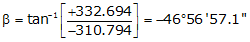Because it's in the SE quadrant: Az = 180°00'00"+(-46°56'57.1") = 133°03'02.9"

Line FG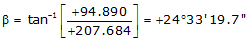Because it's in the NE quadrant: Az = 24°33'19.7"

Line GHBecause it's in the SW quadrant: Az = 180°00'00"+(61°05'18.8") =241°05'18.8"

Line HEBecause it's in the NW quadrant: Az = 360°00'00"+(-10°35'00.5") = 349°24'59.5"